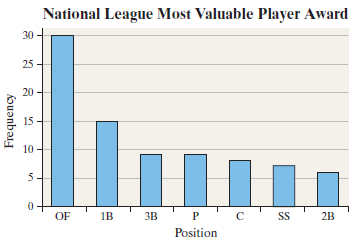×
Get Full Access to Statistics: Informed Decisions Using Data - 5 Edition - Chapter 2.1 - Problem 7
Get Full Access to Statistics: Informed Decisions Using Data - 5 Edition - Chapter 2.1 - Problem 7

×

# ?Most Valuable Player The following Pareto chart shows the position played by the most valuable player (MVP) in the National League since 1931. Source:ISBN: 9780134133539 240

## Solution for problem 7 Chapter 2.1

Statistics: Informed Decisions Using Data | 5th Edition

• Textbook Solutions
• 2901 Step-by-step solutions solved by professors and subject experts
• Get 24/7 help from StudySoup virtual teaching assistantsStatistics: Informed Decisions Using Data | 5th Edition

4 5 1 241 Reviews
31
3
Problem 7

Most Valuable Player The following Pareto chart shows the position played by the most valuable player (MVP) in the National League since 1931. Source: http://www.baseball-almanac.com/(a) Which position had the most MVPs?

(b) How many MVPs played first base (1B)?

(c) How many more MVPs played outfield (OF) than first base?

(d) There are three outfield positions (left field, center field, right field). Given this, how might the graph be misleading?

Step-by-Step Solution:

Step 1 of 5) Most Valuable Player The following Pareto chart shows the position played by the most valuable player (MVP) in the National League since 1931. Source: http://www.baseball-almanac.com/ (a) Which position had the most MVPs (b) How many MVPs played first base (1B) (c) How many more MVPs played outfield (OF) than the first base (d) There are three outfield positions (left field, center field, right field). Given this, how might the graph be misleading

Step 2 of 2

##### ISBN: 9780134133539

Since the solution to 7 from 2.1 chapter was answered, more than 209 students have viewed the full step-by-step answer. The full step-by-step solution to problem: 7 from chapter: 2.1 was answered by , our top Statistics solution expert on 01/15/18, 03:19PM. Statistics: Informed Decisions Using Data was written by and is associated to the ISBN: 9780134133539. The answer to “?Most Valuable Player The following Pareto chart shows the position played by the most valuable player (MVP) in the National League since 1931. Source: http://www.baseball-almanac.com/ (a) Which position had the most MVPs?(b) How many MVPs played first base (1B)?(c) How many more MVPs played outfield (OF) than first base?(d) There are three outfield positions (left field, center field, right field). Given this, how might the graph be misleading?” is broken down into a number of easy to follow steps, and 68 words. This full solution covers the following key subjects: . This expansive textbook survival guide covers 88 chapters, and 2422 solutions. This textbook survival guide was created for the textbook: Statistics: Informed Decisions Using Data, edition: 5.

## Discover and learn what students are asking

Calculus: Early Transcendental Functions : Product and Quotient Rules and Higher-Order Derivatives
?Using the Product Rule In Exercises 1–6, use the Product Rule to find the derivative of the function. $$g(x)=\left(x^{2}+3\right)\left(x^{2}-4 x\ Calculus: Early Transcendental Functions : Inverse Trigonometric Functions: Integration ?In Exercises 1-20, find the indefinite integral. \(\int \frac{1}{x \sqrt{4 x^{2}-1}} d x$$

Statistics: Informed Decisions Using Data : Estimating the Value of a Parameter
?What does the 95% represent in a 95% confidence interval?

Statistics: Informed Decisions Using Data : Comparing Three or More Means (One-Way Analysis of Variance)
?Births by Day of Week An obstetrician knew that there were more live births during the week than on weekends. She wanted to determine whether the mean

Statistics: Informed Decisions Using Data : An Overview of Nonparametric Statistics
?Explain the idea of efficiency.

Unlock Textbook Solution